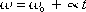omega =   omega-sub-zero   plus    alpha times t

This is one of the constant angular acceleration equations. It's the one with no "theta" in it.

omega [radians-per-second] is the angular velocity of the object at time t.
omega-sub-zero [radians-per-second] is the angular velocity of the object at time 0.
alpha [radians-per-seconds-squared] is the angular acceleration.
t [seconds] is the time.

Tip: In some problems you need to use Newton's Second Law for Rotational Motion, "Torque is equal to I times alpha" to get alpha before you can apply one of the constant angular acceleration equations.

Pitfall Avoidance Note: The units for alpha are not m/s2.